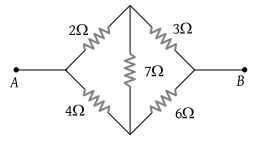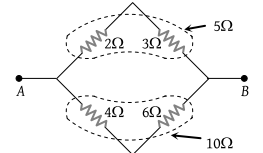NEET Questions Solved

PMT - 1999

Five resistances are connected as shown in the figure. The effective resistance between the points A and B is(1) $\frac{10}{3}\Omega$

(2) $\frac{20}{3}\Omega$

(3) 15 Ω

(4) 6 Ω

(1) The given circuit is a balanced Wheatstone bridge type, hence it can be simplified as follows$⇒{R}_{AB}=\frac{10}{3}\Omega$

Difficulty Level:

• 76%
• 11%
• 9%
• 5%